Top 10 things to know about circles

1. A circle is the set of points in a plane that are equidistant from a given point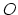called the center.
2. The distance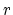from the center is called the radius, and the pointis called the center. Twice the radius is known as the diameter,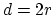.
3. The angle a circle subtends from its center is a full angle, equal to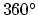or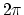radians.
4. The perimeter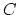of a circle is called the circumference, and is given by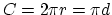.
5. The area of a circle is given by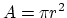.
6. A central angle is an angle with endpoints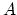andlocated on a circle's circumference and vertexlocated at the circle's center.
7. For an inscribed angle and central angle with the same endpoints,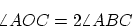8. A tangent to a circle at a point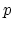is perpendicular to the radius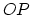.
9. The circumcircle is a triangle's circumscribed circle, i.e., the unique circle that passes through each of the triangle's three vertices. The centerof the circumcircle is called the circumcenter, and the circle's radius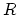is called the circumradius. A triangle's three perpendicular bisectors (i.e. the line perpendicular to the side from the midpoint of the side) meet at.
10. The incircle is the inscribed circle of a triangle , i.e., the unique circle that is tangent to each of the triangle's three sides. The center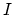of the incircle is called the incenter, and the radiusof the circle is called the inradius. The incenter is the point of concurrence of the triangle's angle bisectors.

This document was generated using the LaTeX2HTML translator Version 2002-2-1 (1.70)

Copyright © 1993, 1994, 1995, 1996, Nikos Drakos, Computer Based Learning Unit, University of Leeds.
Copyright © 1997, 1998, 1999, Ross Moore, Mathematics Department, Macquarie University, Sydney.

The command line arguments were:
latex2html 10_things_circles.tex

The translation was initiated by Steven Dunbar on 2006-02-02Steven R. Dunbar
Department of Mathematics and Statistics